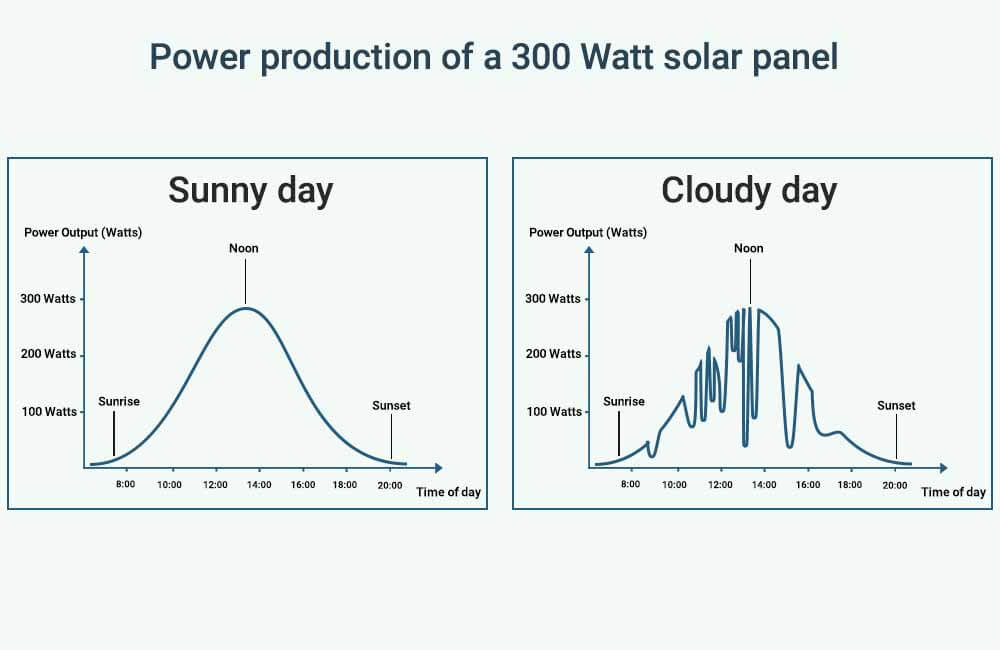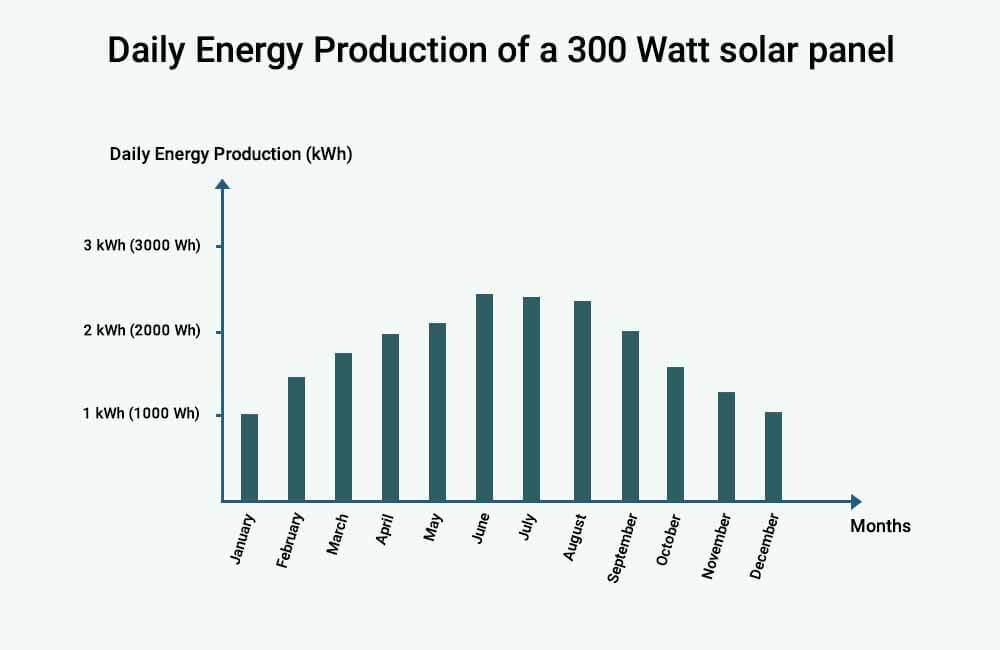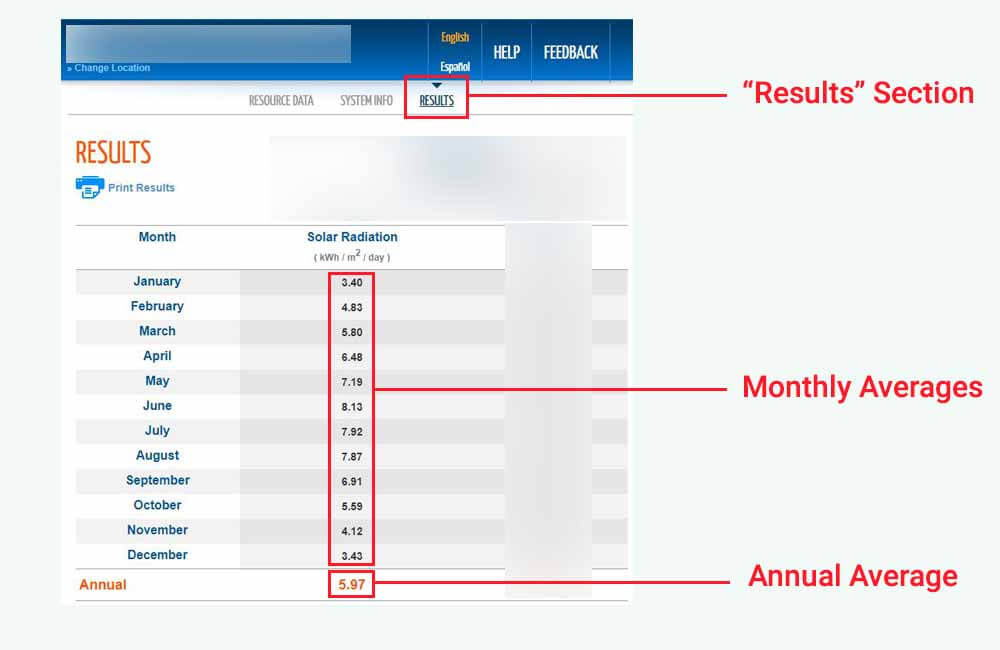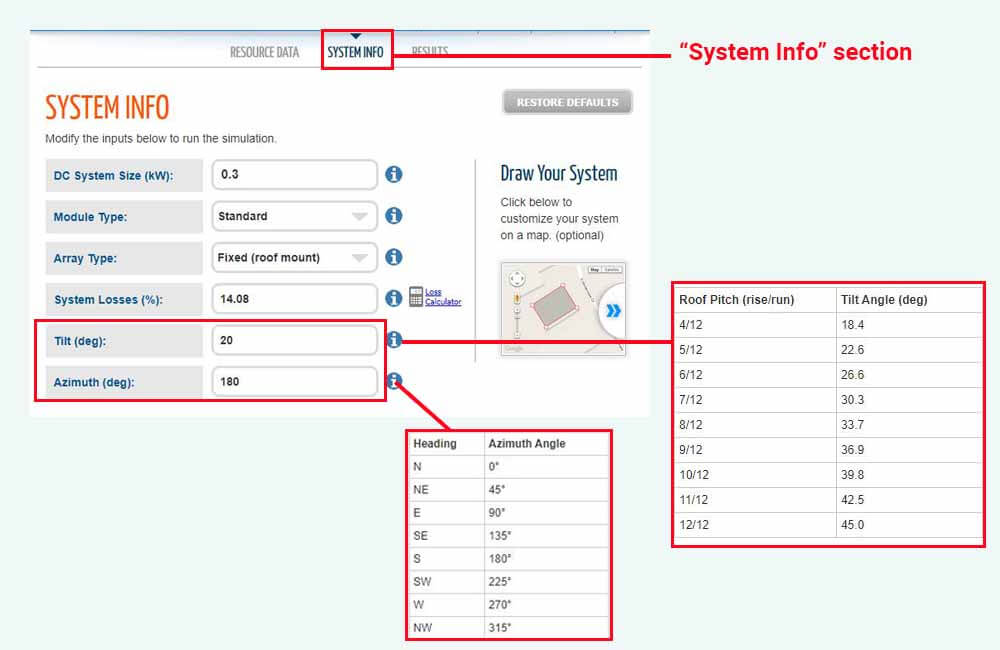# How much power does a 300 Watt solar panel produce?

Solar panels can be a pretty consistent source of energy, but when it comes to exactly how much energy that is, it is important to set your expectations right. To do that, it is important to understand the difference between Power (Watts) and Energy (Watt-hours), and what each of them represents.

Power refers to the rate at which electricity is produced, and Energy refers to the actual quantity of electricity that has been generated.

This article will give you a clearer idea of what that means, and of how much power and energy to expect from a 300 Watt solar panel.

Let’s get started!

## How much power does a 300 Watt solar panel produce?

Solar panels are rated in Watts (W) or kiloWatts (kW), and their Power Output is also measured in Watts or kiloWatts.

Under ideal sunlight conditions, a 300 Watt solar panel has the potential to produce 300 Watts (0.3 kW) of power, or even a little bit more. However, in reality, the power output of a 300 Watt solar panel typically ranges from 100 to 250 Watts (0.1 to 0.25 kW).

But it’s rated at 300 Watts, shouldn’t it produce 300 Watts of power?

The amount of power that a solar panel produces is proportional to the amount of sunlight that it receives. The higher the intensity of the sunlight that a solar panel receives at a given moment, the more power it will produce.

Conversely, the lower the intensity of the sunlight that a solar panel receives, the less power it will produce.

In the real world, the intensity of sunlight is not always consistent, meaning that solar panels will not always produce the full amount of power indicated by their rating (300 Watts in this case).

Let me explain.

In the solar industry, the amount of sunlight hitting a certain surface is measured in Watts per square meter (Watts/m²), and is referred to as “Solar Irradiance”.

The “300 Watt” rating on a solar panel is given after it has been tested under a Solar Irradiance of exactly 1000 Watts/m², as well as other conditions that simulate a perfectly clear, sunny day around noon.

However, it’s not always noon and most days are not perfectly clear and sunny, which means the actual amount of sunlight that a solar panel receives depends on factors such as:

• Time of day
• Season
• Location
• The tilt angle of the solar panel
• The direction that the solar panel is facing
• And of course, weather conditions

For example, the following image shows a comparison of Solar Irradiance between a clear and sunny day and a cloudy day:The fluctuations of Solar Irradiance on a cloudy day are the result of clouds blocking the sun and therefore reducing the intensity of the light hitting the ground.

Similarly, the following image illustrates the comparison of power production of a 300 Watt solar panel on a clear and sunny day versus a cloudy day:However, as I explain in my article about the difference between Power (kW) and Energy (kWh), Power is an instantaneous metric and can vary greatly.

For example, depending on the amount of sunlight available at a given moment, a 300W solar panel might produce 200 Watts of power at one moment and then only 50 Watts a moment later.

Although the instantaneous power production of a solar panel can be an interesting metric, it still is just a snapshot in time and is not the most important factor in determining the effectiveness of the panel.

What truly matters is the overall quantity of electricity, or “Energy (kWh)”, produced over time. It is the more practical measure of a solar panel’s effectiveness, as it reflects the accumulated power production snapshots.

In the next section, I will discuss the Energy production of a 300 Watt solar panel, and how it can be calculated.

## How much energy (kWh) does a 300 Watt solar panel produce?

In the context of solar panels, Energy is measured in Watt-hours (Wh) or more commonly kilo-Watt-hours (kWh), and it represents the quantity of electricity that a solar panel produces over a certain period of time, typically a day.

On average, a 300 Watt solar panel produces between 1.2 and 1.5 kiloWatt-hours (kWh) of energy daily, which translates to 1200 to 1500 Watt-hours (Wh) per day. The energy production of the panel may vary depending on its location and the season, with a range from 0.6 kiloWatt-hours (600 Wh) to 2.5 kiloWatt-hours (2500 Wh).

To illustrate how the location of a solar panel influences its energy production, here’s a table that compares the average daily energy production of a 300 Watt solar panel in different cities in the U.S.:

To illustrate how the energy production of a solar panel fluctuates throughout the year, the following chart displays the average daily energy production of a 300 Watt solar panel in Reno, Nevada over the course of a year:As seen in the chart, the daily energy production of the 300 Watt solar panel fluctuates throughout the year, reaching its peak in the summer months of June and July, and experiencing a drop during the winter months of December and January.

Estimating the daily energy production of a 300 Watt solar panel in your location and for different months can be done using a simple formula:

Daily Energy Production (Watt-hours) = Power Rating of the solar panel (Watts) x Daily Peak Sun Hours in your location

To use this formula, you’ll first need to determine the daily Peak Sun Hours in your location. This refers to the daily amount of solar energy that is available for solar panels to convert into electrical energy in your location.

For example, if you live in a location that – on average – gets 5 Peak Sun Hours a day, the average daily energy production of a 300 Watt solar panel in your location can be calculated as such:

Daily Energy Production (Watt-hours) = Power Rating of the solar panel (Watts) x Daily Peak Sun Hours in your location

Daily Energy Production (Watt-hours) = 300 Watts x 5 Peak Sun Hours

Daily Energy Production (Watt-hours) = 1500 Wh

1500 Wh (Watt-hours) translates to 1.5 kWh (kiloWatt-hours).

So, how can you determine the Peak Sun Hours in your location?

Well, the easiest way to do this would be to use the PVWatts Calculator, a free online tool made available by the U.S. National Renewable Energy Laboratory (NREL).

Based on your address, or the location of the solar panel, the calculator will estimate monthly and annual averages of the daily Peak Sun Hours that your solar panel would receive.

To use the tool, click here and enter the location of the solar panel:Once you’ve submitted a location, go the the “Results” section of the tool:In this section, the calculator provides estimates of the daily Peak Sun Hours for each month, as well as the annual average.

For example, let’s say I want to estimate the daily energy production of the 300 Watt panel in April.

In April, the calculator estimates that this location would – on average – receive 6.48 Peak Sun Hours per day (6.48 kWh/m²/day). The daily energy production of our 300 Watt solar panel could then be calculated as such:

Daily Energy Production (Watt-hours) = Power Rating of the solar panel (Watts) x Daily Peak Sun Hours in your location

Daily Energy Production (Watt-hours) = 300 Watts x 6.48 Peak Sun Hours

Daily Energy Production (Watt-hours) = 1944 Wh

1944 Wh (Watt-hours) translates to about 1.95 kWh (kiloWatt-hours).

It is worth noting that the energy production of the solar panel can vary depending on its tilt angle and orientation. For more accurate estimates, you could go to the “System Info” section of the PVWatts calculator, and modify the default inputs:The “Tilt (deg)” refers to the inclination angle of the solar panel, and the “Azimuth (deg)” input refers to the direction that the solar panel is facing.

For example, if the 300 Watt solar panel is going to be installed on the flat roof of an RV, the tilt angle of the panel is going to be 0 degrees, and the Azimuth angle will not really matter.

If the solar panel is going to be installed on a roof that has a 4/12 Pitch and that is facing due West, the tilt angle of the panel is going to be 18.4 degrees, and the Azimuth angle is going to be 270 degrees.